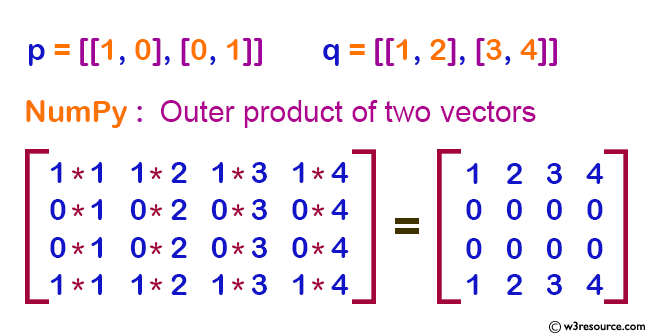﻿ NumPy: Compute the outer product of two given vectors - w3resource# NumPy: Compute the outer product of two given vectors

## NumPy: Linear Algebra Exercise-2 with Solution

Write a NumPy program to compute the outer product of two given vectors.

NumPy: Outer product of two vectorsSample Solution :

Python Code :

``````import numpy as np
p = [[1, 0], [0, 1]]
q = [[1, 2], [3, 4]]
print("original matrix:")
print(p)
print(q)
result = np.outer(p, q)
print("Outer product of the said two vectors:")
print(result)
``````

Sample Output:

```original matrix:
[[1, 0], [0, 1]]
[[1, 2], [3, 4]]
Outer product of the said two vectors:
[[1 2 3 4]
[0 0 0 0]
[0 0 0 0]
[1 2 3 4]]
```

Python Code Editor:

Have another way to solve this solution? Contribute your code (and comments) through Disqus.

What is the difficulty level of this exercise?

Test your Python skills with w3resource's quiz

﻿

## Python: Tips of the Day

Negative Indexing:

In Python you can use negative indexing. While positive index starts with 0, negative index starts with -1.

```name="Welcome"
print(name)
print(name[-1])
print(name[0:3])
print(name[-1:-4:-1])

```

Output:

```W
e
Wel
emo```